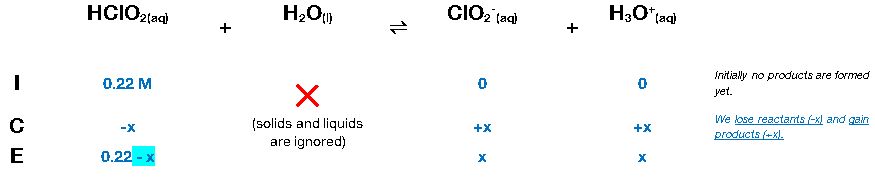# Problem: Calculate the percent dissociation for a 0.22-M solution of chlorous acid (HClO 2, Ka = 1.2 X 10-2).

###### FREE Expert Solution

Given:

Ka = 1.2×10-2

HClO2(aq) + H2O(l)H3O+(aq) + ClO2­-(aq)

We’ll have to construct an ICE chart in order to determine the equilibrium concentration of H3O+.82% (169 ratings)###### Problem Details

Calculate the percent dissociation for a 0.22-M solution of chlorous acid (HClO 2, Ka = 1.2 X 10-2).